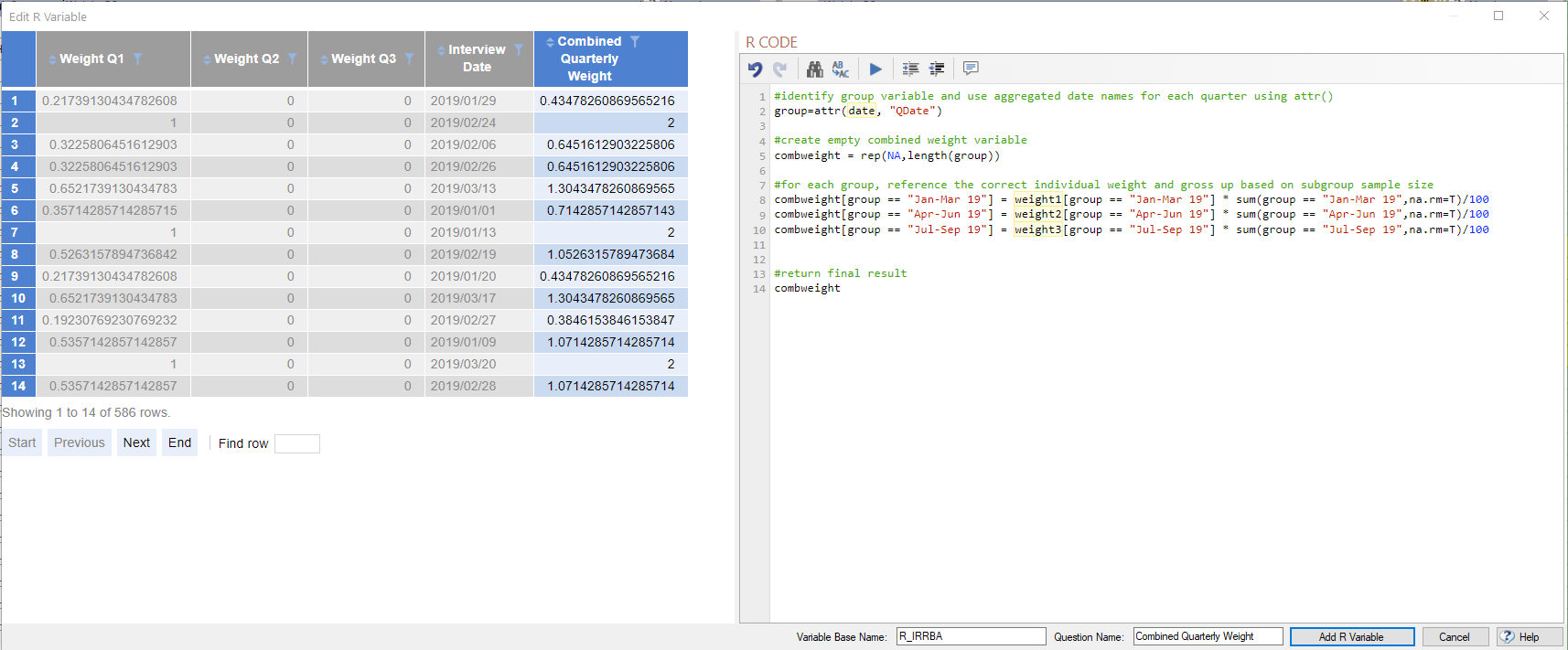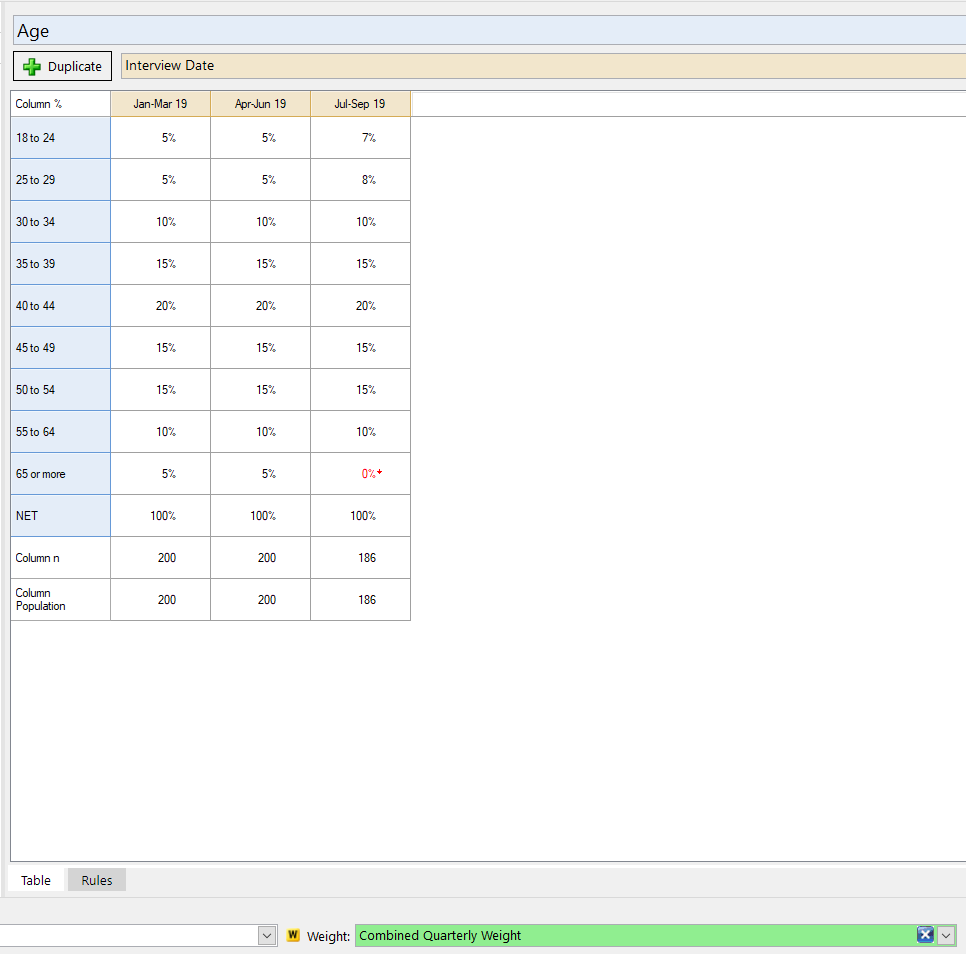# How To Apply Different Weighting Structures To Sub-Samples

If wanting to set different weights to the same target questions/categories within waves, see How To Apply Different Weighting Structures To Different Time Periods. Otherwise, if you need to apply different weights to different target questions or different categories within a sub-sample, please read below. A couple examples of where this comes up:

1. You need to create weights based on country-regions but there are different regions in each country
2. A certain target question category has 0 respondents within a wave

You can manually figure out the population counts or overall weighting percentages and use those in a grid within the weighting tool, but many times that's a little complicated and tedious. The below instructions use the more easily understood target percentages.

## Overall Instructions

1. Setup the targets for the first group (see Construct Weight) where each Weight set has the group variable listed as the Target column question(s) and the remaining groups are given 0 targets
2. Uncheck Force sum of weights to equal sample size for all Weight sets (this will make the Population for each group = 100)
3. Change the Variable Name of the new weight variable to weight1
4. Repeat the above steps for each of the groups
5. Create a JavaScript Variable or R Variable to combined the weights. See example below.
6. Click the W in the Tags column for the new combined weight variable in the Variables and Questions tab

## Combining Weights

If you'd like your groups' populations to be evenly weighted, you can combine the individual weights directly using code similar to below. Replace group with the group variable from your dataset and add/remove groups as needed.

```if (group== 1)
weight1;
else if (group == 2)
weight2;
else if (group == 3)
weight3;
else
weight4;
```

If you'd like your groups' populations to equal the groups sample size within the unweighted data, you can combine the individual weights and gross up/down the respondent-level weights to balance out the sample size. Replace YourGroupVariable on line 2 with the name of the group variable from your dataset, replace 1, 2, 3, etc with each group's label, and add/remove groups as needed.

```# identify group variable
group = YourGroupVariable

#create empty combined weight variable
combweight = rep(NA,length(group))

#for each group, reference the correct individual weight and gross up based on subgroup sample size
combweight[group == "1"] = weight1[group == "1"] * sum(group == "1",na.rm=T)/100
combweight[group == "2"] = weight2[group == "2"] * sum(group == "2",na.rm=T)/100
combweight[group == "3"] = weight3[group == "3"] * sum(group == "3",na.rm=T)/100
combweight[group == "4"] = weight2[group == "4"] * sum(group == "4",na.rm=T)/100

#return final result
combweight
```

## A Worked Example

Weight each quarter of data based on age, but where some quarters don't have all age groups, i.e. Q3 has 0 respondents who are 65 or more.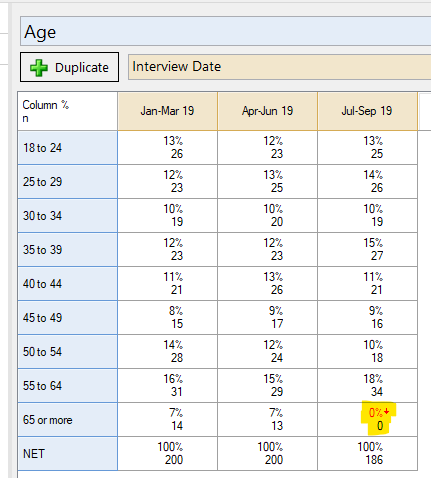Targets within each quarter are as follows: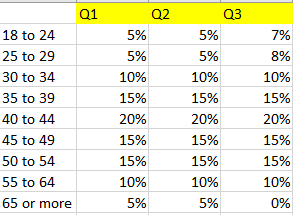We want the final weighted population for each quarter to be equal to the # of respondents in the unweighted data.

To do so:
1. Create the weight for Q1: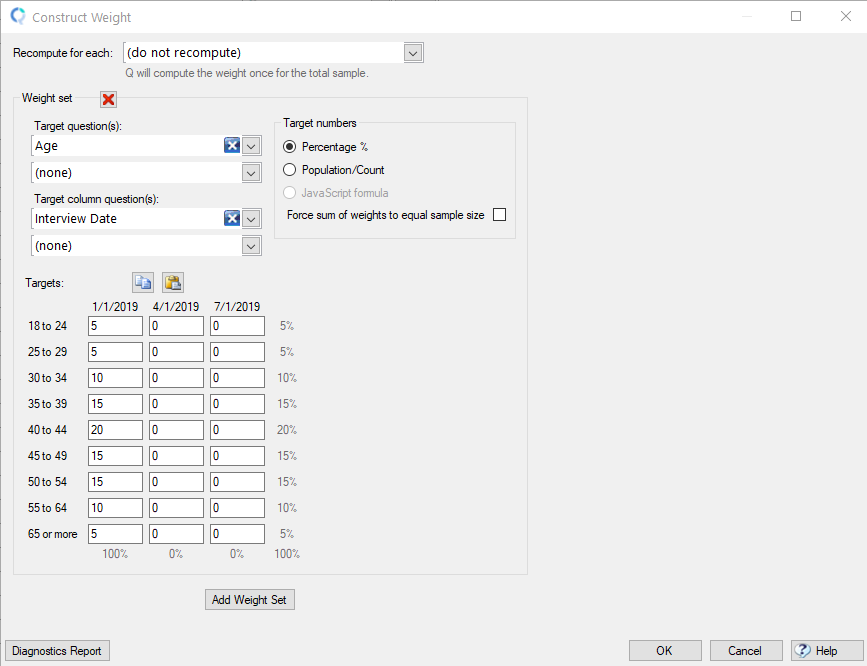2. Create the weight for Q2: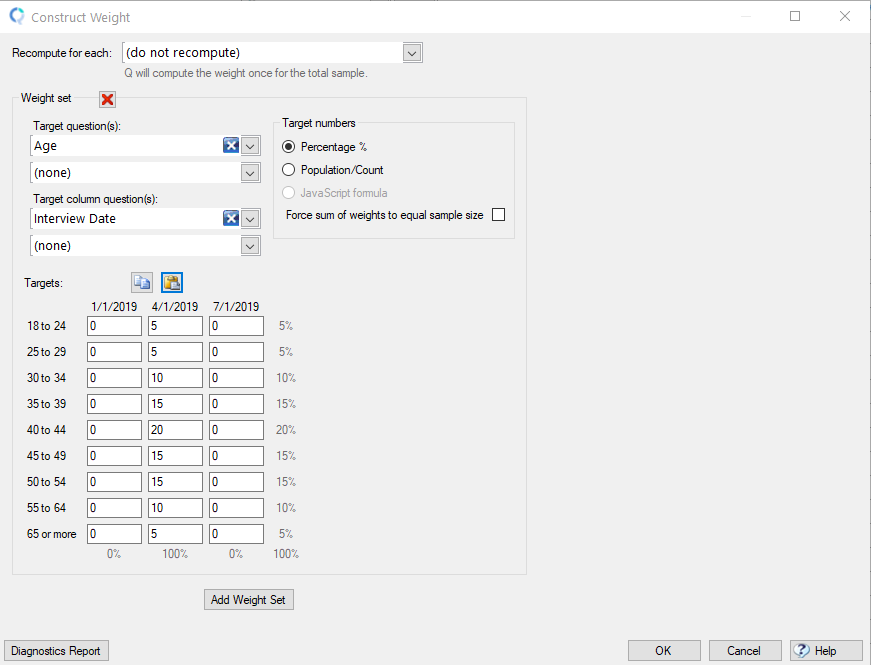3. Create the weight for Q3:4. On the Variable and Questions tab right click on a row and select Insert Variable(s) > R Variable

5. Paste in R CODE similar to below (line 2 has been edited to use the aggregated date values)

```#identify group variable and use aggregated date names for each quarter using attr()
group=attr(date, "QDate")

#create empty combined weight variable
combweight = rep(NA,length(group))

#for each group, reference the correct individual weight and gross up based on subgroup sample size
combweight[group == "Jan-Mar 19"] = weight1[group == "Jan-Mar 19"] * sum(group == "Jan-Mar 19",na.rm=T)/100
combweight[group == "Apr-Jun 19"] = weight2[group == "Apr-Jun 19"] * sum(group == "Apr-Jun 19",na.rm=T)/100
combweight[group == "Jul-Sep 19"] = weight3[group == "Jul-Sep 19"] * sum(group == "Jul-Sep 19",na.rm=T)/100

#return final result
combweight
```

6. Press the blue play button to run the code

7. Give the new variable a Question Name